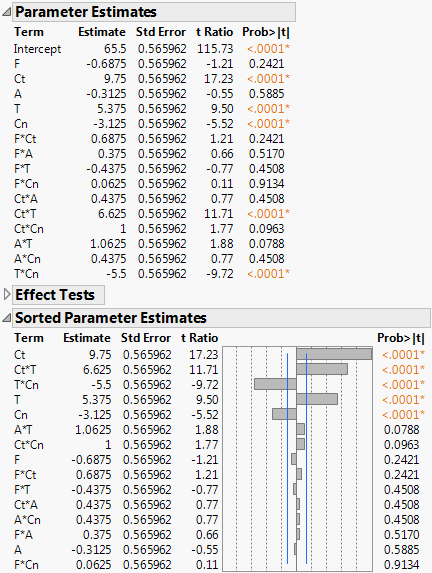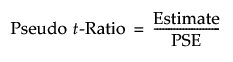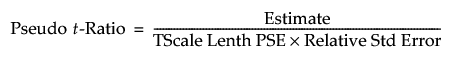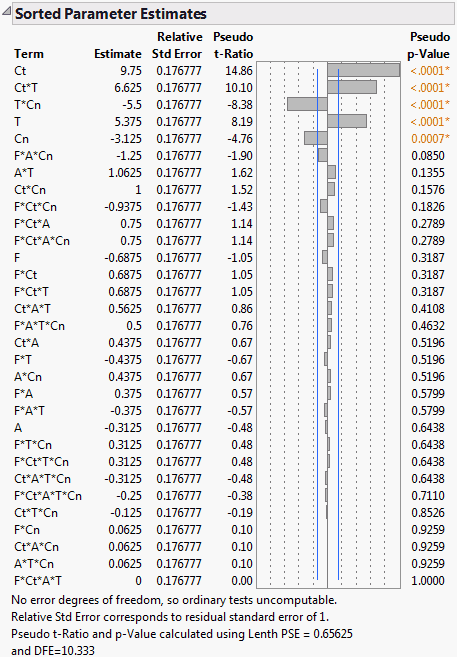Publication date: 11/10/2021

## Sorted Estimates

The Sorted Estimates option produces a version of the Parameter Estimates report that is useful in screening situations. If the design is not saturated, the Sorted Estimates report gives the information found in the Parameter Estimates report, but with the terms, other than the Intercept, sorted in decreasing order of significance (second report in Figure 3.21). If the design is saturated, then Pseudo t tests are provided. These are based on Lenth’s pseudo standard error (Lenth 1989). See Lenth’s PSE.

#### Example of a Sorted Estimates Report

1. Select Help > Sample Data Library and open Reactor.jmp.

2. Select Analyze > Fit Model.

3. Select Y and click Y.

4. Make sure that 2 appears in the Degree box near the bottom of the window.

5. Select F, Ct, A, T, and Cn and click Macros > Factorial to Degree.

6. Click Run.

7. Click the Response Y red triangle and select Estimates > Sorted Estimates.

Figure 3.21 Sorted Parameter EstimatesThe Sorted Parameter Estimates report also appears automatically if the Emphasis is set to Effect Screening and all of the effects have only one parameter.

Note the following differences between the Parameter Estimates report and the Sorted Parameter Estimates report (both shown in Figure 3.21):

The Sorted Parameter Estimates report does not show the intercept.

The effects are sorted by the absolute value of the t ratio, showing the most significant effects at the top.

A bar chart shows the t ratio with vertical lines showing critical values for the 0.05 significance level.

### Sorted Estimates Report for Saturated Models

Screening experiments often involve fully saturated models, where there are not enough degrees of freedom to estimate error. In these cases, the Sorted Estimates report (Figure 3.21) gives relative standard errors and constructs t ratios and p-values using Lenth’s pseudo standard error (PSE). These quantities are labeled with Pseudo in their names. See Lenth’s PSE and Pseudo t-Ratios. A note explains the change and shows the PSE.

The report contains the following columns:

Term

The model term whose coefficient is of interest.

Estimate

The parameter estimates are presented in sorted order, with smallest p-values listed first.

Relative Standard Error

If there are no degrees of freedom for residual error, the report gives relative standard errors. The relative standard error is computed by setting the root mean square error equal to 1.

Pseudo t-Ratio

A t ratio for the estimate, computed using pseudo standard error. The value of Lenth PSE is shown in a note at the bottom of the report.

Pseudo p-Value

A p-value computed using an error degrees of freedom value (DFE) of m/3, where m is the number of parameters other than the intercept. The value of DFE is shown in a note at the bottom of the report.

#### Lenth’s PSE

Lenth’s pseudo standard error (PSE) is an estimate of residual error due to Lenth (1989). It is based on the principle of effect sparsity: in a screening experiment, relatively few effects are active. The inactive effects represent random noise and form the basis for Lenth’s estimate.

The value is computed as follows:

1. Consider the absolute values of all non-intercept parameters.

2. Remove all parameter estimates whose absolute values exceed 3.75 times the median absolute estimate.

3. Multiply the median of the remaining absolute values of parameter estimates by 1.5.

#### Pseudo t-Ratios

When relative standard errors are equal, Lenth’s PSE is shown in a note at the bottom of the report. The Pseudo t-Ratio is calculated as follows:When relative standard errors are not equal, the TScale Lenth PSE is computed. This value is the PSE of the estimates divided by their relative standard errors. The Pseudo t-Ratio is calculated as follows:Note that, to estimate the standard error for a given estimate, TScale Lenth PSE is adjusted by multiplying it by the estimate’s relative standard error.

#### Example of a Saturated Model

1. Select Help > Sample Data Library and open Reactor.jmp.

2. Select Analyze > Fit Model.

3. Select Y and click Y.

4. Select the following five columns: F, Ct, A, T, and Cn.

5. Click the Macros button and select Full Factorial.

6. Click Run.

7. Click the Response Y red triangle and select Estimates > Sorted Estimates.

Note that Lenth’s PSE and the degrees of freedom used are given at the bottom of the report. The report indicates that, based on their Pseudo p-Values, the effects Ct, Ct*T, T*Cn, T, and Cn are highly significant.

Figure 3.22 Sorted Parameter Estimates Report for Saturated Model